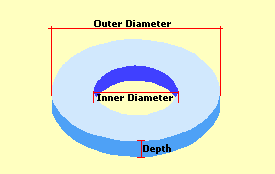Spike's Calculators

# Donut Shape Footing or Slab

Calculate the amount of concrete you need in cubic feet and yards for a footing or slab in the shape of a donut.
Note: this is a circular area with a circular hole on the inside, not a torus!!

To calculate the concrete volume needed for this type of slab, you need to know the outer diameter of the circle, the inner diameter, and the required slab depth.### Formula's

##### Total Area
```A = π * D²  ÷ 4
where A is the area in square feet
π = 3.14159265358979
D = outer diameter of the circle```
##### Perimeter
```P = π * D ÷
where P is the perimeter in feet
p = 3.14159265358979
D = diameter of the circle```
##### Volume
```V = π * D² ÷ 4 * (d ÷ 12) where V is the volume in cubic feet
p = 3.14159265358979
D = diameter of circle
d = depth of slab in inches```
##### Example calculation for volume:
```outer diameter = 24 feet
inner diameter = 22 feet
depth of slab = 4 inches
```
Calculate the total volume first, then the inside area volume, and subtract this from the total volume;
`3.14159265358979 * 24 * 24 ÷ 4 * ( 4 ÷ 12) = 150.796`
`The donut's total volume is 150.796 cubic feet.`

The inner area's volume;
`3.14159265358979 * 22 * 22 ÷ 4 * ( 4 ÷ 12) = 126.711`
`The volume of the inner circle is 126.711cubic feet`

Subtract the inner volume from the total volume:
`150.796 - 126.711 = 24.1`
`You need 24.1 cubic feet of concrete for this footing.`

To convert cubic feet to cubic yards multiply cubic feet * 0.037037037037037
To convert cubic feet to cubic metres multiply cubic feet * 0.028316846592
To convert cubic yards to cubic metres multiply cubic yards * 0.764554857984

### Donut Shaped Slab / Footing

Outer Diameter ft in
Inner Diameter ft in
Depth in

Width of Footing in
Surface Area of Footing ft²
Inner Perimeter ft
Outer Perimeter ft
Total Length of Perimeter ft

Cubic Feet ft ³
Cubic Yards yd ³
Cubic Metres

#### Calculation

1. enter the outer diameter in feet and inches
2. enter the inner diameter in feet and inches
3. the depth in inches

#### Results

1. footing or slab width in inches
2. square footage of this area. (the surface area of the footing or slab)
3. inside perimeter in lineal feet
4. outside perimeter in lineal feet
5. combined perimeter the outside plus the inside in lineal feet
6. amount of concrete needed in cubic feet
7. concrete needed in cubic yards
8. concrete needed in cubic metres

### When Ordering Concrete

This concrete calculator will help you in estimating the amount of concrete needed for your project. The amount given as needed, does not include any waste. It is recommended, depending on the job you are doing, to add anywhere from an extra 4% to 10% to your concrete order to make sure you have enough concrete to finish the job. Ask when ordering the concrete. They will be able to give you a good idea of what is needed.